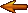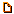# Topic: PHP seems to "forget" changes made to an array in a loop. (Page 1 of 1)DavidJCobb
Nervous Wreck (II) Inmate

From: United States
Insane since: Mar 2009posted 04-09-2009 23:26

I'm trying to make a code for a game site that will convert strings resembling the following...

code:
```*Legend of Zelda: Ocarina of Time
**Index
**Glitches
***Steal the Fishing Rod
**Videos
**Guides
**Secrets
**Cheats```

Into an array structure resembling the following:

code:
```array(
'Legend of Zelda: Ocarina of Time'=>
array(
'Index'=>array(),
'Glitches'=>
array(
array(
),
'Steal the Fishing Rod'=>array()
),
'Videos'=>array(),
'Guides'=>array(),
'Secrets'=>array(),
'Cheats'=>array()
)
);```

Now, to do this, I explode the string at each newline (\$treeData = explode("\n", \$myString)) and use a foreach to loop through the array of lines. I process each line, using them to build another array, \$tree. Basically, once that foreach loop ends, \$tree reverts back to the value it had before any of the looping happened.

My code:

code:
```<pre><?php
function OutputTreeView(\$treeString,\$root=0) {
\$treeData=explode("\n",\$treeString); // array of lines
\$tree = array();
\$curs = array();
\$offset = 0; // not important
if(\$root) { // FROM HERE TO THE NEXT COMMENT... IS NOT IMPORTANT.
\$line=\$treeData;
\$offset=strspn(\$line,'*');
\$level=0;
\$tree[substr(\$line,\$level+\$offset)] = array();
\$curs[\$level]=substr(\$line,\$level+\$offset);
array_shift(\$treeData);
} else {
\$level=0;
\$line='';
\$tree['[root]']=array();
\$curs[\$level]='[root]';
} // FROM HERE TO THE PREVIOUS COMMENT IS NOT IMPORTANT.
foreach(\$treeData as \$line) {
\$level= strspn(\$line,'*')-\$offset; // use the "*"s at the beginning to determine how nested we are.
\$curs[\$level]=substr(\$line,\$level+\$offset); // used to keep track of where we are in \$tree, so that we can build \$varString
\$varString = '\$tree['; // prepare for variable substitution (the double dollar sign trick)
for(\$i=0;\$i<=\$level;\$i++) {
\$varString.="'".\$curs[\$i]."']";
if(\$i<\$level) { \$varString.='['; }
}
\$\$varString = array(); // the actual variable substitution, used to alter \$tree
// [debug statement removed]
if(\$level+1<count(\$curs)) { // this IF and the WHILE inside of it is only NEEDED for debugging.
while(count(\$curs)>\$level+1) { array_pop(\$curs); }
}
}
return \$tree;
}

\$TREE='*Legend of Zelda: Ocarina of Time'."\n".'**Index'."\n".
'***Steal the Fishing Rod'."\n".'**Videos'."\n".'**Guides'."\n".
'**Secrets'."\n".'**Cheats';
// The string example I presented above. I woulda used a nowdoc,
// but PHP was throwing a T_SL error even though there was nothing
// wrong with the syntax (I Googled the error to figure out the cause,
// and the cause wasn't present) -- ah, well.

echo OutputTreeView(\$TREE);
?></pre>```

Using a large amount of debug statements (most of which have been removed to shorten the code), I am able to tell that \$tree is modified during the loop, but these modifications don't "stick" at the end of the loop. Now, \$tree isn't the array I'm looping through, so AFAIK I don't need to place an ampersand before it ("&\$tree" shouldn't be necessary)... Plus a debug statement after the loop, checking \$\$varString, shows the modifications that should be visible just by checking \$tree normally...

And some debug output that'll probably help to clarify what my code actually does:

code:
```Function invoked!
\$treeString ==
*Legend of Zelda: Ocarina of Time
**Index
**Glitches
***Steal the Fishing Rod
**Videos
**Guides
**Secrets
**Cheats

\$root == 1 so "*Legend of Zelda: Ocarina of Time" is the "root" of this list, and \$offset = 1.

Iteration! \$level==1, \$line==**Index,
\$varString==\$tree['Legend of Zelda: Ocarina of Time']['Index']
[here, we set \$\$varString = array()]
\$\$varString==Array
Iteration! \$level==1, \$line==**Glitches,
\$varString==\$tree['Legend of Zelda: Ocarina of Time']['Glitches']
[here, we set \$\$varString = array()]
\$\$varString==Array
\$varString==\$tree['Legend of Zelda: Ocarina of Time']['Glitches']['Swordless Link']
[here, we set \$\$varString = array()]
\$\$varString==Array
[here, we set \$\$varString = array()]
\$\$varString==Array
Iteration! \$level==2, \$line==***Steal the Fishing Rod,
\$varString==\$tree['Legend of Zelda: Ocarina of Time']['Glitches']['Steal the Fishing Rod']
[here, we set \$\$varString = array()]
\$\$varString==Array
Iteration! \$level==1, \$line==**Videos,
\$varString==\$tree['Legend of Zelda: Ocarina of Time']['Videos']
[here, we set \$\$varString = array()]
\$\$varString==Array
Iteration! \$level==1, \$line==**Guides,
\$varString==\$tree['Legend of Zelda: Ocarina of Time']['Guides']
[here, we set \$\$varString = array()]
\$\$varString==Array
Iteration! \$level==1, \$line==**Secrets,
\$varString==\$tree['Legend of Zelda: Ocarina of Time']['Secrets']
[here, we set \$\$varString = array()]
\$\$varString==Array
Iteration! \$level==1, \$line==**Cheats,
\$varString==\$tree['Legend of Zelda: Ocarina of Time']['Cheats']
[here, we set \$\$varString = array()]
\$\$varString==Array

Function complete.

And when we print_r \$tree, we see:
(
[Legend of Zelda: Ocarina of Time] => Array
(
)

)
...Which is weird, because \$tree['Legend of Zelda: Ocarina of Time'] shouldn't be empty.

And when we print_r \$\$varString, we see:
Array
(
)
Which makes sense.

And when we print_r \$curs, we see:
Array
(
 => Legend of Zelda: Ocarina of Time
 => Cheats
)```

I am thoroughly confused.

----------------------(Edited by DavidJCobb on 04-09-2009 23:28)

Tyberius Prime
Maniac (V) Mad Scientist with Finglongers

From: Germany
Insane since: Sep 2001posted 04-10-2009 08:55

I will bet even money that your \$\$ magic doesn't do what you think it does.
And you don't really need it.
Rework your code to live without it (use recursion, much cleaner).

here, from the top of my head, untested...

code:
```function createTree(\$lines, \$depth = 1)
{
if (!is_array(\$lines))
\$lines = split("\n", lines);

\$tree = array();
while (count(\$lines) > 0 )
{
\$nextLine = array_unshift(\$lines);
\$newDepth = strspn(\$nextLine,'*');
\$lineName = substr(\$nextLine, \$newDepth);
if (\$newDepth == \$depth)
{
\$tree[\$lineName] = array();
\$lastName = &\$lineName;
}
else
{
\$tree[\$lineName] = createTree(\$lines, \$depth + 1);
}
return \$tree;
}```

so long,

->Tyberius Prime